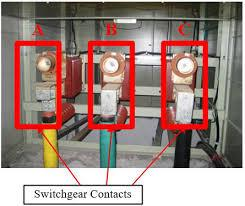# Quiz On Circuit Breaker

15 Questions | Total Attempts: 60Settings.

• 1.
The medium employed for extinction of arc in air circuit breaker is
• A.

(A) SF6

• B.

(B) Oil

• C.

(C) Air

• D.

(D) Water.

• 2.
Which of the following circuit breakers is preferred for EHT application
• A.

(A) Air blast circuit breakers

• B.

(B) Minimum oil circuit breakers

• C.

(C) Bulk oil circuit breakers

• D.

(D) SF6 oil circuit breakers.

• 3.
For high voltage, ac circuit breakers, the rated short circuit current is passed for
• A.

(A) 0.01 sec

• B.

(B) 0.1 sec

• C.

(C) 3 seconds

• D.

(D) 30 seconds.

• 4.
Interrupting medium in a contactor may be
• A.

(A) air

• B.

(B) oil

• C.

(C) SF6 gas

• D.

(D) any of the above.

• 5.
In air blast circuit breakers, the pressure of air is of the order of
• A.

(A) 100 mm Hg

• B.

(B) 1 kg/cm2

• C.

(C) 20 to 30 kg/cm2

• D.

(D) 200 to 300 kg/cm2

• 6.
SF6 gas is
• A.

(A) sulphur fluoride

• B.

(B) sulphur difluoride

• C.

(C) sulphur hexafluorine

• D.

(D) sulphur hexafluoride.

• 7.
SFgas
• A.

(A) is lighter than hydrogen

• B.

(B) is lighter than air

• C.

(C) has density 2-times as compared to that of air

• D.

(D) has density 5 limes as compared to that of air.

• 8.
The pressure of SF6 gas in circuit breakers is of the order of
• A.

(A) 100 mm Hg

• B.

(B) 1 kg/cm2

• C.

(C) 3 to 5 kg/cm2

• D.

(D) 30 to 50 kg/cm2.

• 9.
While selecting a gas for circuit breaker, the property of gas that should be considered is
• A.

(A) high dielectric strength

• B.

(B) non-inflammability

• C.

(C) non-toxicity

• D.

(D) all of the above.

• 10.
A sudden short-circuit in an ac system causes a rise in __________ in the short-circuited phase.
• 11.
The voltage appearing across the circuit breaker pole after final current zero is called __________ voltage.
• 12.
The voltage across the circuit breaker pole after final current zero is recovery voltage.
• A.

True

• B.

False

• 13.
Air circuit breakers are used for voltages above 12 kV.
• A.

True

• B.

False

• 14.
Arcing contacts of circuit-breakers are generally made of copper tungsten alloy.
• A.

True

• B.

False

• 15.
The insulation requirements of switchgear are determined by __________ voltage.Back to top